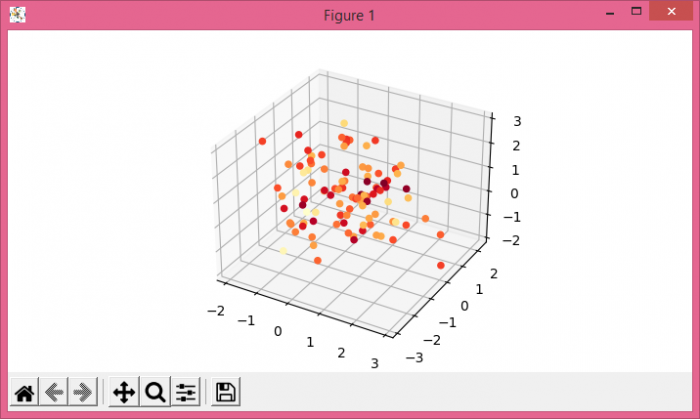# How to make a 4D plot with Matplotlib using arbitrary data?

To make a 4D plot, we can create x, y, z and c standard data points. Create a new figure or activate an existing figure.

## Steps

• Use figure() method to create a figure or activate an existing figure.

• Add a figure as part of a subplot arrangement.

• Create x, y, z and c data points using numpy.

• Create a scatter plot using scatter method.

• To display the figure, use show() method.

## Example

from matplotlib import pyplot as plt
import numpy as np
plt.rcParams["figure.figsize"] = [7.00, 3.50]
plt.rcParams["figure.autolayout"] = True
fig = plt.figure()
plt.show()